# Neural Automated Model Discovery for Autocompleting Models with Prior Structural Information

When calibrating models to data we can have uncertainties about the parameter values, but also about the structure of the model. It could happen that no matter how we try to fit the data, there are no good enough parametrizations, which might point out that we need to adjust the equations of the model.

This is what Universal Differential Equations (UDEs for short) try to solve. Starting from the model and the data that you have, we find the minimal mechanistic extension that would provide a better fit. In this tutorial, we will show how to use the JuliaSimModelOptimizer to extend a partially correct model and auto-complete it to find the missing physics.

The model that we will consider will be the Lotka-Volterra equations. These equations are given by:

\begin{aligned} \frac{dx}{dt} &= \alpha x - \beta x y \\ \frac{dy}{dt} &= -\delta y + \gamma x y \\ \end{aligned}

This is a model of rabbits and wolves. $\alpha x$ is the exponential growth of rabbits in isolation, $-\beta x y$ and $\gamma x y$ are the interaction effects of wolves eating rabbits, and $-\delta y$ is the term for how wolves die hungry in isolation.

Now assume that we have never seen rabbits and wolves in the same room. We only know the two effects $\alpha x$ and $-\delta y$. Can we use Scientific Machine Learning to automatically discover an extension to what we already know? That is what we will solve with the universal differential equation.

## First steps

For this tutorial we will need the following packages:

ModuleDescription
JuliaSimModelOptimizerThe high-level library used to formulate our problem and perform automated model discovery
ModelingToolkitThe symbolic modeling environment
OrdinaryDiffEq (DifferentialEquations.jl)The numerical differential equation solvers
CSV and DataFramesWe will read our experimental data from .csv files
DataSetsWe will load our experimental data from datasets on JuliaHub
OptimizationOptimisersThe optimization solver package with Adam
OptimizationOptimJLThe optimization solver package with LBFGS
DataDrivenSparseThe sparse regression symbolic regression solvers
PlotsThe plotting and visualization library
StableRNGsStable random seeding

Besides that we'll also need the following Julia standard libraries:

ModuleDescription
LinearAlgebraRequired for the norm function
StatisticsRequired for the mean function
using JuliaSimModelOptimizer
using ModelingToolkit
using OrdinaryDiffEq
using CSV, DataFrames
using DataSets
using OptimizationOptimJL: LBFGS
using Plots
using StableRNGs
using LinearAlgebra
using Statistics
Plots.GRBackend()

## Problem setup

We will now define the incomplete model using ModelingToolkit and read in the training data (that corresponds to the correct model data + some small normally distributed noise).

function incomplete_lotka()
iv = only(@variables(t))
sts = @variables x(t)=5.0 y(t)=5.0
ps = @parameters α=1.3 β=0.9 γ=0.8 δ=1.8
∂ = Differential(t)

eqs = [
∂(x) ~ α * x,
∂(y) ~ - δ * y,
]

return ODESystem(eqs,
iv,
sts,
ps;
name = :lotka)
end

rng = StableRNG(1111)

training_dataset = dataset("juliasimtutorials/lotka_data")
data = open(IO, training_dataset) do io
end

scatter(data.timestamp, [data."x(t)" data."y(t)"], color = :red, label = ["Noisy Data" nothing])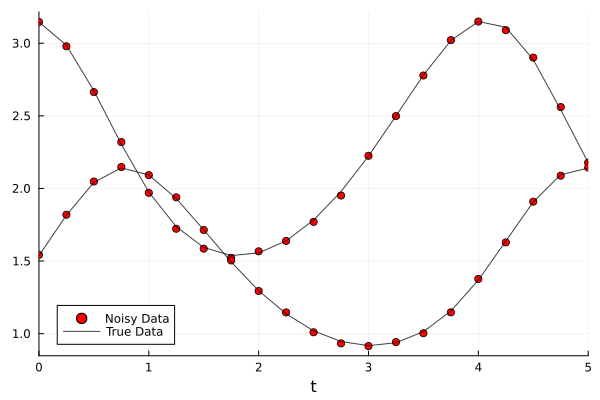## Training the neural network

We will now train the neural network by calibrating its parameters to the training data.

# We use the incomplete model in the experiment definition
incomplete_model = complete(incomplete_lotka())
@unpack x, y = incomplete_model
experiment = Experiment(data, incomplete_model, alg = Vern7(), abstol = 1e-6, reltol = 1e-6, u0=[x=>data."x(t)", y=>data."y(t)"])
Experiment
with initial conditions:
x(t) => 3.1463924566781167
y(t) => 1.5423300037202512.
timespan: (0.0, 5.0)


In order to add a neural network to the output of the model, we specify the neural network to be used via the neural_network keyword argument.

The neural_network keyword argument accepts a Lux.jl model. For ease of use, the JuliaSimModelOptimizer provides functions that can build such models easily, such as multi_layer_feed_forward. As our model has 2 states, the input and output of the neural network will be 2.

prob = InverseProblem(experiment, incomplete_model, []; neural_network = multi_layer_feed_forward(2, 2), nn_rng=rng)
InverseProblem for lotka with one experiment optimizing 0 parameters.


We now train the neural network by calibrating the inverse problem in two stages:

r1 = calibrate(prob, SingleShooting(; maxiters = 5000, optimizer = Adam()))
Calibration result computed in 3 minutes and 45 seconds. Final objective value: 28.314.
Optimization ended with Default Return Code.



We now use this optimization result as an initial guess for a second optimization using LBFGS

r2 = calibrate(r1, SingleShooting(; maxiters = 1000, optimizer = LBFGS()))
Calibration result computed in 2 minutes and 23 seconds. Final objective value: 0.00269927.
Optimization ended with Failure Return Code.



## Visualizing the training results

Let's visualize how well was the neural network trained:

pl_losses = convergenceplot([r1, r2], yscale=:log10)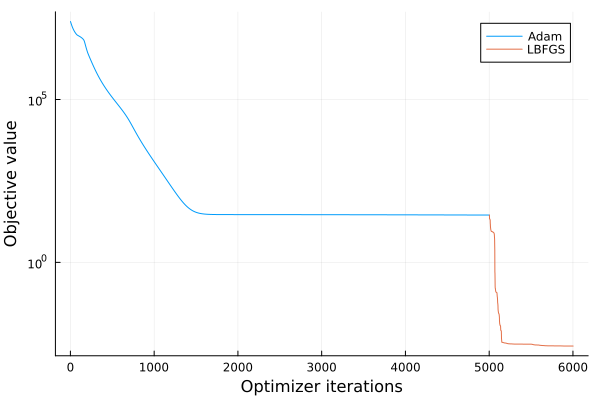Next, we compare the original data to the output of the UDE predictor.

pl_trajectory = plot(experiment, prob, r2, show_data=true, legend=:bottomleft)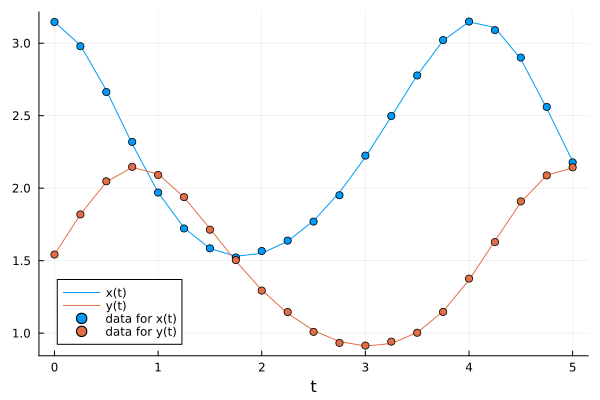Let's see how well the unknown term has been approximated:

X̂ = simulate(experiment, prob, r2)
β = ModelingToolkit.defaults(incomplete_model)[incomplete_model.β]
γ = ModelingToolkit.defaults(incomplete_model)[incomplete_model.γ]
# Ideal unknown interactions of the predictor
Ȳ = [-β * (X̂[1, :] .* X̂[2, :])'; γ * (X̂[1, :] .* X̂[2, :])']
# Neural network guess
Ŷ = network_prediction(prob)(X̂, r2)

ts = X̂.t

pl_reconstruction = plot(ts, transpose(Ŷ), xlabel = "t", ylabel = "U(x,y)", color = :red, label = ["UDE Approximation" nothing])
plot!(ts, transpose(Ȳ), color = :black, label = ["True Interaction" nothing])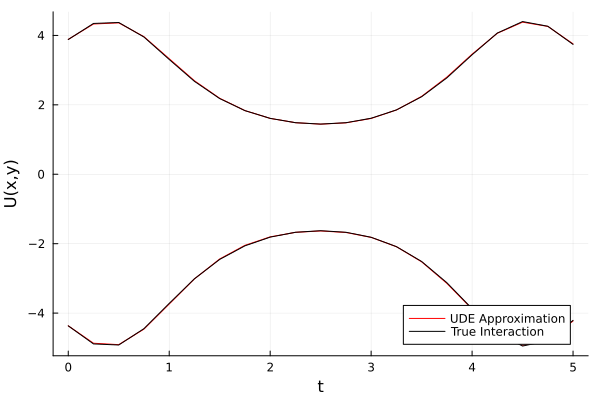and we can also take a look at the reconstruction error

# Plot the error
pl_reconstruction_error = plot(ts, norm.(eachcol(Ȳ - Ŷ)), yaxis = :log, xlabel = "t", ylabel = "L2-Error", label = nothing, color = :red)
pl_missing = plot(pl_reconstruction, pl_reconstruction_error, layout = (2, 1))

pl_overall = plot(pl_trajectory, pl_missing)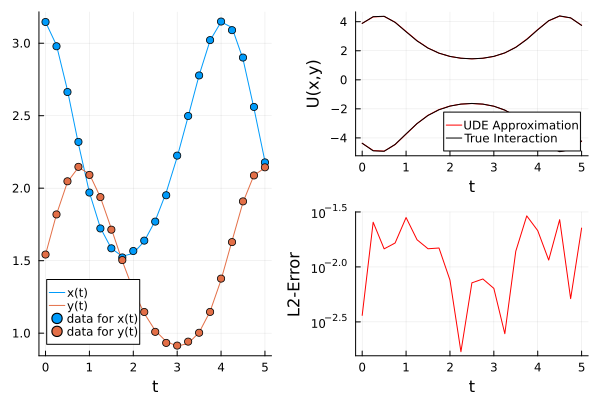## Symbolic interpretation

We can now use the training results to autocomplete our model with symbolic terms that match the data. This can be done with the autocomplete function, which is compatible with DataDrivenDiffEq algorithms. For this example we will use the ADMM algorithm from DataDrivenSparse and we will assum that our missing terms come from a polynomial basis of degree 4. In order to improve the accuracy of the symbolic interpretation, we process the neural network predictions in batches and we normalize the results using the Z-score transformation.

λ = exp10.(-3:0.01:3)
data_processing = DataProcessing(split = 0.9, batchsize = 30, shuffle = true)
res = autocomplete(r2, alg;
basis_generator = (polynomial_basis, 4),
digits = 1,
normalize = DataNormalization(ZScoreTransform),
data_processing)
Calibration result computed in 17 seconds and 835 milliseconds. Final objective value: 0.00373857.
Optimization ended with Success Return Code and returned.

┌─────────────┬───────────┬──────────┐
│          p₁ │        p₂ │       p₃ │
├─────────────┼───────────┼──────────┤
│ -0.00576501 │ -0.896935 │ 0.800489 │
└─────────────┴───────────┴──────────┘


The autocomplete function returns a calibration result as the new model parameters are calibrated to best match the data. We can extract the autocompleted model using get_model.

get_model(res)
\begin{align} \frac{\mathrm{d} x\left( t \right)}{\mathrm{d}t} =& p_1 + \alpha x\left( t \right) + p_2 x\left( t \right) y\left( t \right) \\ \frac{\mathrm{d} y\left( t \right)}{\mathrm{d}t} =& - \delta y\left( t \right) + p_3 x\left( t \right) y\left( t \right) \end{align}

In order to evaluate how good is our new model, we can try making predictions outside of the training data by solving for a larger timespan.

ex = only(get_experiments(res))
plot(ex, res, tspan=(0,50.), saveat=0.1, show_data=true, legend=:topright)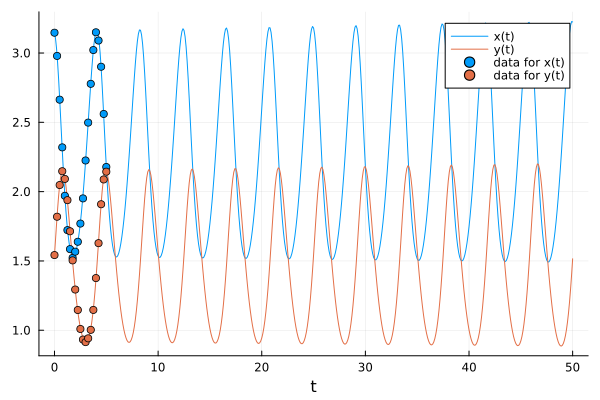• 1Derived from https://docs.sciml.ai/Overview/stable/showcase/missing_physics/ from https://github.com/SciML/SciMLDocs, MIT licensed, see repository for details